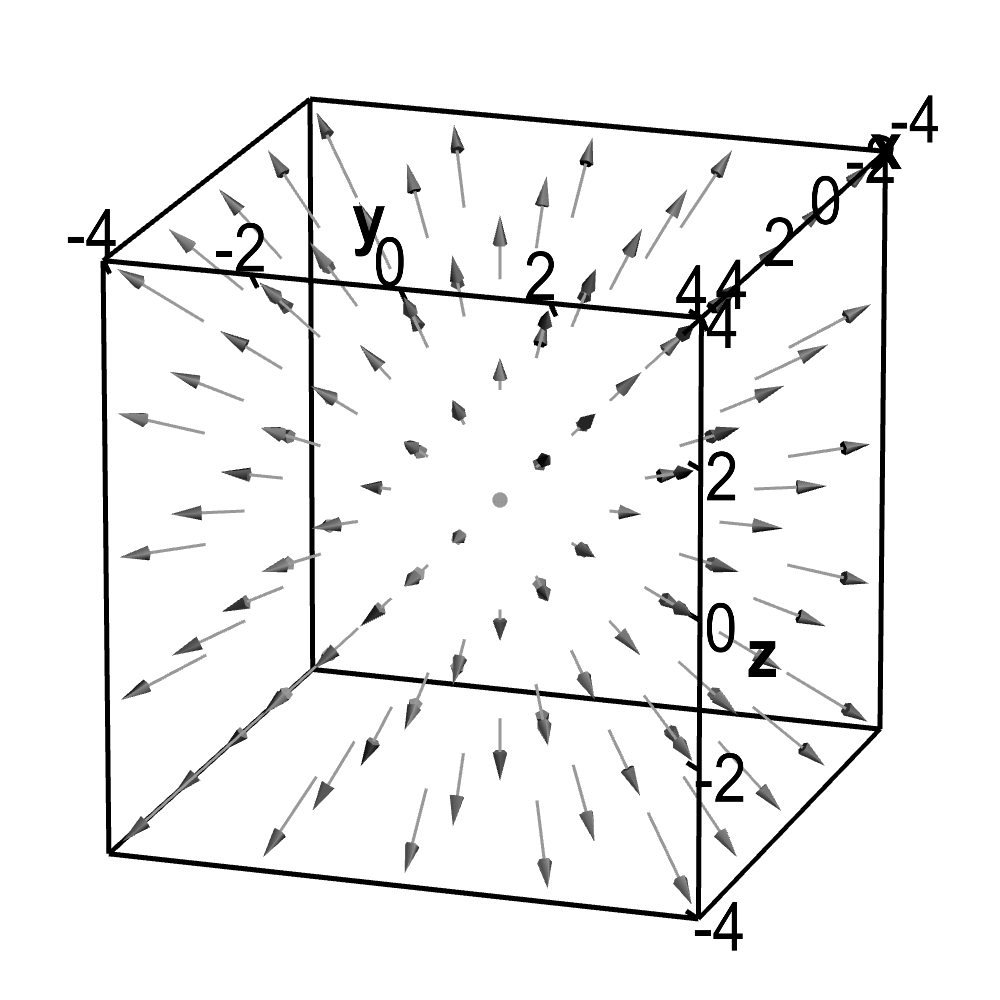# Math Insight

### Applet: Divergent vector fieldThis vector field illustrates a uniform expansion of fluid and is a simple example of a field with positive divergence. The vector field is $\dlvf(x,y,z) = (x,y,z)$. Its divergence is $\pdiff{\dlvfc_1}{x}+\pdiff{\dlvfc_2}{y}+\pdiff{\dlvfc_3}{z}=1+1+1=3$.